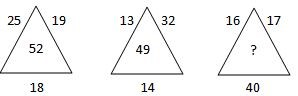# Group 1 Prelims 2013 February TNPSC Question Paper

## Group 1 Prelims 2013 February TNPSC Questions

21.

Match the following:

 List I List II a) Karukar 1.) Thaiyalkarar (Tailor) b) Karodar 2.) Aranmanaikkavalar (Palace Security) c) Yavanar 3.) Nesavuthozhilalar (Weaver) d) Thunnakkarar 4.) Sanai Pitippavar
A.

3 4 2 1

B.

4 3 2 1

C.

2 4 3 1

D.

1 2 3 4

A. 3 4 2 1

22.

Critically observe the reaction and choose the correct RQ from the options given below:

C18H34O2 + 25.5O2 --> 18CO2 + 17 H2O

The correct RQ of this reaction is

A.

0.071

B.

0.71

C.

7.1

D.

71

B. 0.71

23.

Which of the following statements is/are not relevant to Gandhi-Irwin pact?

I. Government agreed to withdraw the repressive ordinance.

II. Government agreed to release satyagraha prisoners.

III. Congress agreed to participate in the third round table conference.

IV. Gandhi agreed to suspend the civil disobedience movement.

A.

II and III

B.

I only

C.

II and IV

D.

III only

D. III only

24.
The average marks in English obtained by 300 students is 45. The average marks of first 100 and last 100 students are 70 and 20 respectively. The average marks of remaining 100 students is
A.
70
B.
20
C.
45
D.
50
C. 45
25.
Struges rule for determining the number of classes (K) in a frequency table is
A.
K = 1 + 3.32log10N
B.
K = 1 - 3.32log10N
C.
K = 1 + 2.32log10N
D.
K = 1 + 3.32logeN
A. K = 1 + 3.32log10N
26.

Consider the following five processes with the length of the CPU burst time given in milliseconds together with their arrival time and priority.

Process       Arrival time      Burst time     Priority

P1                    0                        10               4

P2                    0                         3                1

P3                    3                         8                2

P4                    4                        16               4

P5                    7                          2               5

In priority scheduling the average turn around time will be

A.

20.6 ms

B.

18.6 ms

C.

21.6 ms

D.

22.5 ms

A. 20.6 ms

27.

What should come in the place of question mark (?)A.

63

B.

73

C.

53

D.

83

A. 63

28.

Horizontal bar diagram is used for

A.

Qualitative data

B.

Data varying over time

C.

Data varying over space

D.

(A) or (C)

D. (A) or (C)

29.
Classification is the process of arranging data in
A.
Different columns
B.
Different rows
C.
Grouping of related facts in different classes
D.
None of these